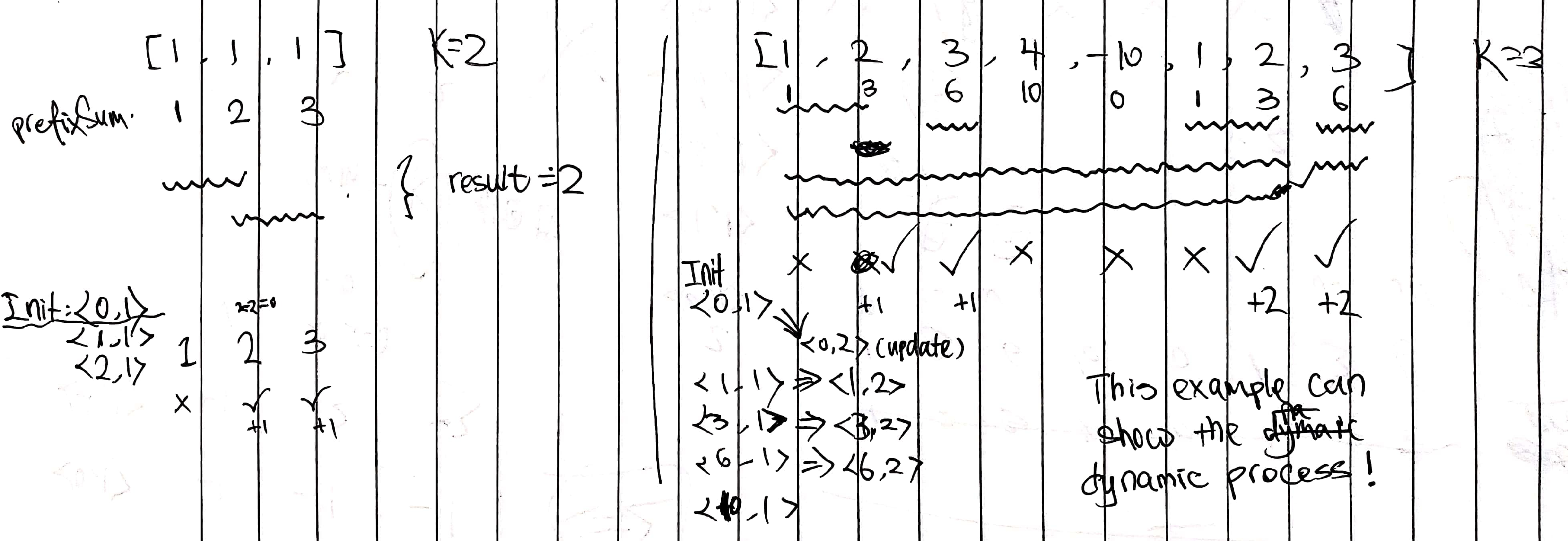# 560. Subarray Sum Equals K

Reference: LeetCode
Difficulty: Medium

## Problem

Given an array of integers and an integer k, you need to find the total number of continuous subarrays whose sum equals to k.

Example:

Note:

• The length of the array is in range [1, 20,000].
• The range of numbers in the array is [-1000, 1000] and the range of the integer k is [-1e7, 1e7].

Follow up: Can you reduce the time complexity to $O(N)$?

## Analysis

### Brute-Force

Time: $O(N^3)$
Space: $O(1)$

### Cummulative Sum

The idea is based on sum(start, end) = sum(0, end) - sum(0, start).

Time: $O(N^2)$
Space: $O(N)$

Improvement:

Actually, we can do it without extra space. The following code iterate through each possible subarray. We notice that we can accumulate the sum in each step.

Time: $O(N^2)$
Space: $O(1)$

### Hash Map

Intuition:

First, let’s assume k = 0. We denote sum[i] be the sum of elements in [0, i], which is like a prefix sum.

For sum[i] and sum[j] (i <= j), if they are equal, we would say the sum of elements at i+1, i+2, ..., j between i and j is 0.

Generally, we can have the difference between sum[i] and sum[j] is k, so the the sum of these elements in between is k.

Based on this idea, we can write the code, but it is not easy. Consider the following:

• How do we use a map? <prefixSum, count>?
• Why do we need map.put(0, 1)?

Consider the examples:Note: Difficult

• Why should we do it in one pass and update count in map dynamically?
• Why do we use sum - k instead of sum + k?

Time: $O(N)$
Space: $O(N)$

CommentJunhao Wang
a software engineering cat